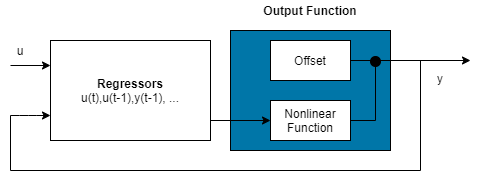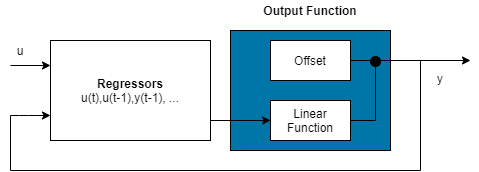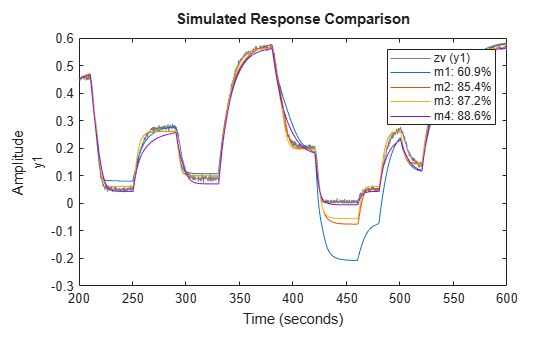Documentation

Estimate Nonlinear ARX Models at the Command Line

You can estimate nonlinear ARX models after you perform the following tasks:

Estimate Model Using nlarx

Use nlarx to both construct and estimate a nonlinear ARX model. After each estimation, validate the model by comparing it to other models and simulating or predicting the model response.

Basic Estimation

Start with the simplest estimation using m = nlarx(data,[na nb nk]). For example:

% na = nb = 2 and nk = 1
m = nlarx(z1,[2 2 1])
m =
Nonlinear ARX model with 1 output and 1 input
Inputs: u1
Outputs: y1
Standard regressors corresponding to the orders:
na = 2, nb = 2, nk = 1
No custom regressor
Nonlinear regressors:
y1(t-1)
y1(t-2)
u1(t-1)
u1(t-2)
Nonlinearity: wavenet with 1 unit

Sample time: 0.1 seconds

Status:
Estimated using NLARX on time domain data "z1".
Fit to estimation data: 68.83% (prediction focus)
FPE: 1.975, MSE: 1.885

The second input argument [na nb nk] specify the model orders and delays. By default, the nonlinearity estimator is the wavelet network (see the wavenet reference page), which takes all standard regressors as inputs to its linear and nonlinear functions. m is an idnlarx object.

For MIMO systems, nb, nf, and nk are ny-by-nu matrices. See the nlarx reference page for more information about MIMO estimation.

Specify a different nonlinearity estimator (for example, sigmoid network).

M = nlarx(z1,[2 2 1],'sigmoid');

Create an nlarxOptions option set and configure the Focus property to minimize simulation error.

opt = nlarxOptions('Focus','simulation');
M = nlarx(z1,[2 2 1],'sigmoid',opt);

Configure Model Regressors

Standard Regressors

Change the model order to create a model structure with different model regressors, which are delayed input and output variables that are inputs to the nonlinearity estimator.

Custom Regressors

Explore including custom regressors in the nonlinear ARX model structure. Custom regressors are in addition to the standard model regressors (see Nonlinear ARX Model Orders and Delay).

Use polyreg or customreg to construct custom regressors in terms of model input-output variables. You can specify custom regressors using the CustomRegressors property of the idnlarx class or addreg to append custom regressors to an existing model.

For example, generate regressors as polynomial functions of inputs and outputs:

m = nlarx(z1,[2 2 1],'sigmoidnet');
getreg(m) % displays all regressors
Regressors:
y1(t-1)
y1(t-2)
u1(t-1)
u1(t-2)
% Generate polynomial regressors up to order 2:
reg = polyreg(m)
4x1 array of Custom Regressors with fields: Function, Arguments, Delays, Vectorized

Append polynomial regressors to CustomRegressors.

getreg(m)
Regressors:
y1(t-1)
y1(t-2)
u1(t-1)
u1(t-2)
y1(t-1).^2
y1(t-2).^2
u1(t-1).^2
u1(t-2).^2

m now includes polynomial regressors.

You can also specify arbitrary functions of input and output variables. For example:

m = nlarx(z1,[2 2 1],'sigmoidnet','CustomReg',{'y1(t-1)^2','y1(t-2)*u1(t-3)'});
getreg(m) % displays all regressors
Regressors:
y1(t-1)
y1(t-2)
u1(t-1)
u1(t-2)
y1(t-1)^2
y1(t-2)*u1(t-3)

Append polynomial regressors to CustomRegressors.

getreg(m) % polynomial regressors
Regressors:
y1(t-1)
y1(t-2)
u1(t-1)
u1(t-2)
y1(t-1)^2
y1(t-2)*u1(t-3)
y1(t-1).^2
y1(t-2).^2
u1(t-1).^2
u1(t-2).^2

Manipulate custom regressors using the CustomRegressors property of the idnlarx class. For example, to get the function handle of the first custom regressor in the array:

CReg1 = m.CustomReg(1).Function;

View the regressor expression.

m.CustomReg(1).Display
ans =
'y1(t-1)^2'

You can exclude all standard regressors and use only custom regressors in the model structure by setting na=nb=nk=0:

m = nlarx(z1,[0 0 0],'linear','CustomReg',{'y1(t-1)^2','y1(t-2)*u1(t-3)'});

In advanced applications, you can specify advanced estimation options for nonlinearity estimators. For example, wavenet and treepartition classes provide the Options property for setting such estimation options.

Specify Linear and Nonlinear Regressors

By default, all model regressors enter as inputs to both linear and nonlinear function blocks of the nonlinearity estimator. To reduce model complexity and keep the estimation well-conditioned, use a subset of regressors as inputs to the nonlinear function of the nonlinear estimator block. For example, specify a nonlinear ARX model to be linear in past outputs and nonlinear in past inputs.

m = nlarx(z1,[2 2 1]); % all standard regressors are
% inputs to the nonlinear function
getreg(m); % lists all standard regressors
Regressors:
y1(t-1)
y1(t-2)
u1(t-1)
u1(t-2)
m = nlarx(z1,[4 4 1],sigmoidnet,'nlreg',[5 6 7 8]);

This example uses getreg to determine the index of each regressor from the complete list of all model regressors. Only regressor numbers 5 through 8 are inputs to the nonlinear function - getreg shows that these regressors are functions of the input variable u1. nlreg is an abbreviation for the NonlinearRegressors property of the idnlarx class. Alternatively, include only input regressors in the nonlinear function block using:

m = nlarx(z1,[4 4 1],sigmoidnet,'nlreg','input');

When you are not sure which regressors to include as inputs to the nonlinear function block, specify to search during estimation for the optimum regressor combination:

m = nlarx(z1,[4 4 1],sigmoidnet,'nlreg','search');

This search typically takes a long time. You can display the search progress using:

opt = nlarxOptions('Display','on');
m = nlarx(z1,[4 4 1],sigmoidnet,'nlreg','search',opt);

After estimation, use m.NonlinearRegressors to view which regressors were selected by the automatic regressor search.

m.NonlinearRegressors
ans = 1×4

3     5     6     7

Configure Nonlinearity Estimator

Specify the nonlinearity estimator directly in the estimation command as:

• A character vector of the nonlinearity name, which uses the default nonlinearity configuration.

m = nlarx(z1, [2 2 1],'sigmoidnet');
• Nonlinearity object.

m = nlarx(z1,[2 2 1],wavenet('num',5));

This estimation uses a nonlinear ARX model with a wavelet nonlinearity that has 5 units. To construct the nonlinearity object before providing it as an input to the nonlinearity estimator:

w = wavenet('num', 5);
m = nlarx(z1,[2 2 1],w);
% or
w = wavenet;
w.NumberOfUnits = 5;
m = nlarx(z1,[2 2 1],w);

For MIMO systems, you can specify a different nonlinearity for each output. For example, to specify sigmoidnet for the first output and wavenet for the second output:

data = [z1, z2(1:300)];
M = nlarx(data,[[1 1;1 1] [2 1;1 1] [2 1;1 1]],[sigmoidnet wavenet]);

If you want the same nonlinearity for all output channels, specify one nonlinearity.

M = nlarx(data,[[1 1;1 1] [2 1;1 1] [2 1;1 1]],sigmoidnet);

The following table summarizes values that specify nonlinearity estimators.

NonlinearityValue (Default Nonlinearity Configuration)Class
Wavelet network
(default)
'wavenet' or 'wave'wavenet
One layer sigmoid network'sigmoidnet' or 'sigm'sigmoidnet
Tree partition'treepartition' or 'tree'treepartition
F is linear in x'linear' or [ ]linear

Additional available nonlinearities include multilayered neural networks and custom networks that you create.

Specify a multilayered neural network using:

m = nlarx(data,[na nb nk],NNet)

where NNet is the neural network object you create using the Deep Learning Toolbox™ software. See the neuralnet reference page.

Specify a custom network by defining a function called gaussunit.m, as described in the customnet reference page. Define the custom network object CNetw and estimate the model:

CNetw = customnet(@gaussunit);
m = nlarx(data,[na nb nk],CNetw)

Exclude Linear Function in Nonlinearity Estimator

If your model includes wavenet, sigmoidnet, and customnet nonlinearity estimators, you can exclude the linear function using the LinearTerm property of the nonlinearity estimator. The nonlinearity estimator becomes F(x)=$g\left(Q\left(x-r\right)\right)$.For example:

SNL = sigmoidnet('LinearTerm','off');
m = nlarx(z1,[2 2 1],SNL);

Note

You cannot exclude the linear function from tree partition and neural network nonlinearity estimators.

Exclude Nonlinear Function in Nonlinearity Estimator

Configure the nonlinear ARX structure to include only the linear function in the nonlinearity estimator by setting the nonlinearity to linear. In this case, F(x)=${L}^{T}\left(x\right)+d$ is a weighted sum of model regressors plus an offset. Such models provide a bridge between purely linear ARX models and fully flexible nonlinear models.In the simplest case, a model with only standard regressors is linear (affine). For example, this structure:

m = nlarx(z1,[2 2 1],'linear');

is similar to the linear ARX model:

lin_m = arx(z1,[2 2 1]);

However, the nonlinear ARX model m is more flexible than the linear ARX model lin_m because it contains the offset term, d. This offset term provides the additional flexibility of capturing signal offsets, which is not available in linear models.

A popular nonlinear ARX configuration in many applications uses polynomial regressors to model system nonlinearities. In such cases, the system is considered to be a linear combination of products of (delayed) input and output variables. Use the polyreg command to easily generate combinations of regressor products and powers.

For example, suppose that you know the output y(t) of a system to be a linear combination of (y(t − 1))2 and y(t − 2)*u(t − 3). To model such a system, use:

M = nlarx(z1,[0 0 0],'linear','CustomReg',{'y1(t-1)^2','y1(t-2)*u1(t-3)'});

M has no standard regressors and the nonlinearity in the model is described only by the custom regressors.

Iteratively Refine Model

If your model structure includes nonlinearities that support iterative search (see Specify Estimation Options for Nonlinear ARX Models), you can use nlarx to refine model parameters:

m1 = nlarx(z1,[2 2 1],'sigmoidnet');
m2 = nlarx(z1,m1); % can repeatedly run this command

You can also use pem to refine the original model:

m2 = pem(z1,m1);

Check the search termination criterion m.Report.Termination.WhyStop . If WhyStop indicates that the estimation reached the maximum number of iterations, try repeating the estimation and possibly specifying a larger value for the nlarxOptions.SearchOptions.MaxIterations estimation option:

opt = nlarxOptions;
opt.SearchOptions.MaxIterations = 30;
m2 = nlarx(z1,m1,opt); % runs 30 more iterations
% starting from m1

When the m.Report.Termination.WhyStop value is Near (local) minimum, (norm( g) < tol or No improvement along the search direction with line search , validate your model to see if this model adequately fits the data. If not, the solution might be stuck in a local minimum of the cost-function surface. Try adjusting the SearchOptions.Tolerance value or the SearchMethod option in the nlarxOptions option set, and repeat the estimation.

You can also try perturbing the parameters of the last model using init (called randomization) and refining the model using nlarx:

M1 = nlarx(z1, [2 2 1], 'sigm'); % original model
M1p = init(M1);	% randomly perturbs parameters about nominal values
M2 = nlarx(z1, M1p);  % estimates parameters of perturbed model

You can display the progress of the iterative search in the MATLAB Command Window using the nlarxOptions.Display estimation option:

opt = nlarxOptions('Display','on');
M2= nlarx(z1,M1p,opt);

Troubleshoot Estimation

If you do not get a satisfactory model after many trials with various model structures and algorithm settings, it is possible that the data is poor. For example, your data might be missing important input or output variables and does not sufficiently cover all the operating points of the system.

Nonlinear black-box system identification usually requires more data than linear model identification to gain enough information about the system.

Use nlarx to Estimate Nonlinear ARX Models

This example shows how to use nlarx to estimate a nonlinear ARX model for measured input/output data.

Prepare the data for estimation.

z = iddata(y, u, 0.2);
ze = z(1:1000); zv = z(1001:3000);

Estimate several models using different model orders, delays, and nonlinearity settings.

m1 = nlarx(ze,[2 2 1]);
m2 = nlarx(ze,[2 2 3]);
m3 = nlarx(ze,[2 2 3],wavenet('num',8));
m4 = nlarx(ze,[2 2 3],wavenet('num',8),...
'nlr', [1 2]);

An alternative way to perform the estimation is to configure the model structure first, and then to estimate this model.

m5 = idnlarx([2 2 3],sigmoidnet('num',14),'nlr',[1 2]);
m5 = nlarx(ze,m5);

Compare the resulting models by plotting the model outputs with the measured output.

compare(zv, m1,m2,m3,m4,m5)